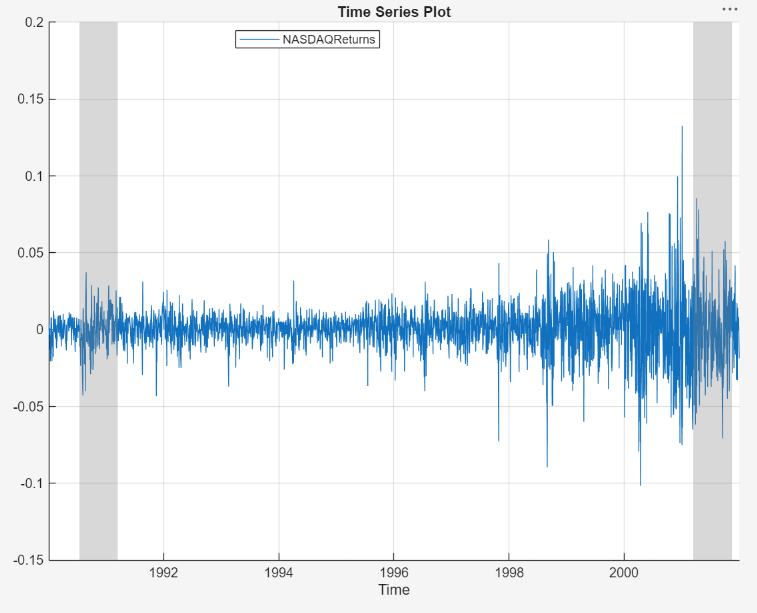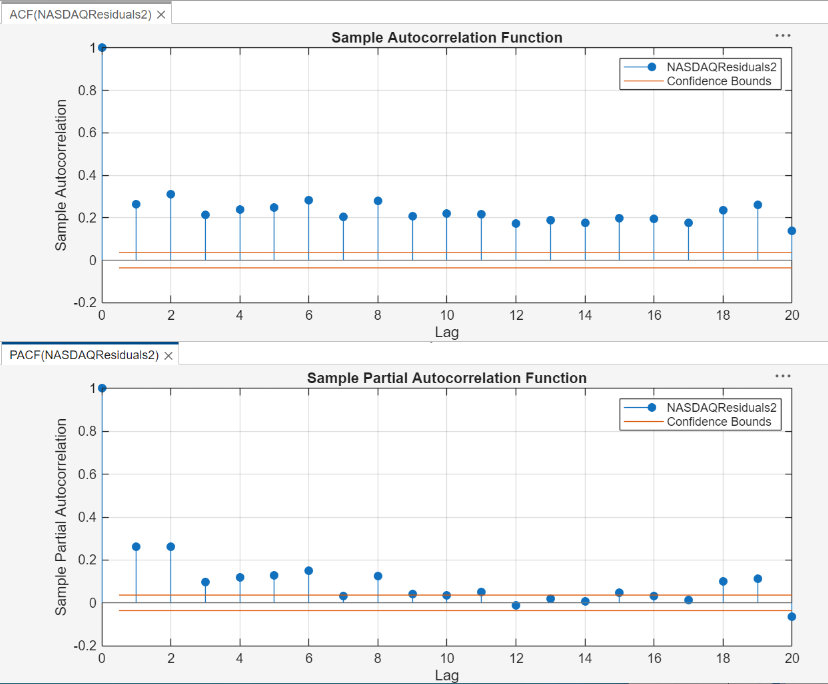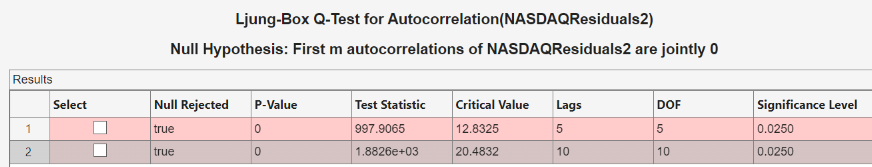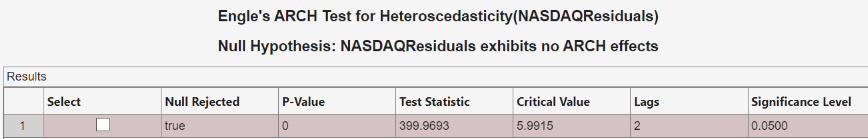## Detect ARCH Effects Using Econometric Modeler App

These examples show how to assess whether a series has volatility clustering by using the Econometric Modeler app. Methods include inspecting correlograms of squared residuals and testing for significant ARCH lags. The data set, stored in `Data_EquityIdx.mat`, contains a series of daily NASDAQ closing prices from 1990 through 2001.

### Inspect Correlograms of Squared Residuals for ARCH Effects

This example shows how to visually determine whether a series has significant ARCH effects by plotting the autocorrelation function (ACF) and partial autocorrelation function (PACF) of a series of squared residuals.

At the command line, load the `Data_EquityIdx.mat` data set.

`load Data_EquityIdx`

The data set contains a table of NASDAQ and NYSE closing prices, among other variables. For more details about the data set, enter `Description` at the command line.

Convert the table `DataTable` to a timetable (for details, see Prepare Time Series Data for Econometric Modeler App).

```dates = datetime(dates,'ConvertFrom','datenum',... 'Format','ddMMMyyyy'); % Convert dates to datetimes DataTable.Properties.RowNames = {}; % Clear row names DataTable = table2timetable(DataTable,'RowTimes',dates); % Convert table to timetable```

At the command line, open the Econometric Modeler app.

`econometricModeler`

Alternatively, open the app from the apps gallery (see Econometric Modeler).

Import `DataTable` into the app:

1. On the Econometric Modeler tab, in the Import section, click.

2. In the Import Data dialog box, in the Import? column, select the check box for the `DataTable` variable.

3. Click .

The variables appear in the Data Browser, and a time series plot of all the series appears in the Time Series Plot(NASDAQ) figure window.

Convert the daily close NASDAQ index series to a percentage return series by taking the log of the series, then taking the first difference of the logged series:

1. In the Data Browser, select `NASDAQ`.

2. On the Econometric Modeler tab, in the Transforms section, click .

3. With `NASDAQLog` selected, in the Transforms section, click .

4. Change the name of `NASDAQLogDiff` to `NASDAQReturns`:

1. In the Data Browser, right-click `NASDAQLogDiff`.

2. In the context menu, select Rename.

3. Enter `NASDAQReturns`.

The time series plot of the NASDAQ returns appears in the Time Series Plot(NASDAQReturns) figure window.The returns appear to fluctuate around a constant level, but exhibit volatility clustering. Large changes in the returns tend to cluster together, and small changes tend to cluster together. That is, the series exhibits conditional heteroscedasticity.

Compute squared residuals:

1. Export `NASDAQReturns` to the MATLAB® Workspace:

1. In the Data Browser, right-click `NASDAQReturns`.

2. In the context menu, select Export.

`NASDAQReturns` appears in the MATLAB Workspace.

2. At the command line:

1. For numerical stability, scale the returns by a factor of 100.

2. Create a residual series by removing the mean from the scaled returns series. Because you took the first difference of the NASDAQ prices to create the returns, the first element of the returns is missing. Therefore, to estimate the sample mean of the series, you must use `nanmean`.

3. Square the residuals.

4. Add the squared residuals as a new variable to the `DataTable` timetable.

```NASDAQReturns = 100*NASDAQReturns; NASDAQResiduals = NASDAQReturns - nanmean(NASDAQReturns); NASDAQResiduals2 = NASDAQResiduals.^2; DataTable.NASDAQResiduals2 = NASDAQResiduals2;```

In Econometric Modeler, import `DataTable`:

1. On the Econometric Modeler tab, in the Import section, click.

2. In the Econometric Modeler dialog box, click to clear all variables and documents in the app.

3. In the Import Data dialog box, in the Import? column, select the check box for `DataTable`.

4. Click .

Plot the ACF and PACF:

1. In the Data Browser, select the `NASDAQResiduals2` time series.

2. Click the Plots tab, then click .

3. Click the Plots tab, then click .

4. Close the Time Series Plot(NASDAQ) figure window. Then, position the ACF(NASDAQResiduals2) figure window above the PACF(NASDAQResiduals2) figure window.The sample ACF and PACF show significant autocorrelation in the squared residuals. This result indicates that volatility clustering is present.

### Conduct Ljung-Box Q-Test on Squared Residuals

This example shows how to test squared residuals for significant ARCH effects using the Ljung-Box Q-test.

At the command line:

1. Load the `Data_EquityIdx.mat` data set.

2. Convert the NASDAQ prices to returns. To maintain the correct time base, prepend the resulting returns with a `NaN` value.

3. Scale the NASDAQ returns.

4. Compute residuals by removing the mean from the scaled returns.

5. Square the residuals.

6. Add the vector of squared residuals as a variable to `DataTable`.

7. Convert `DataTable` from a table to a timetable.

For more details on the steps, see Inspect Correlograms of Squared Residuals for ARCH Effects.

```load Data_EquityIdx NASDAQReturns = 100*price2ret(DataTable.NASDAQ); NASDAQReturns = [NaN; NASDAQReturns]; NASDAQResiduals2 = (NASDAQReturns - nanmean(NASDAQReturns)).^2; DataTable.NASDAQResiduals2 = NASDAQResiduals2; dates = datetime(dates,'ConvertFrom','datenum'); DataTable.Properties.RowNames = {}; DataTable = table2timetable(DataTable,'RowTimes',dates);```

At the command line, open the Econometric Modeler app.

`econometricModeler`

Alternatively, open the app from the apps gallery (see Econometric Modeler).

Import `DataTable` into the app:

1. On the Econometric Modeler tab, in the Import section, click.

2. In the Import Data dialog box, in the Import? column, select the check box for the `DataTable` variable.

3. Click .

The variables appear in the Data Browser, and a time series plot of all the series appears in the Time Series Plot(NASDAQ) figure window.

Test the null hypothesis that the first m = 5 autocorrelation lags of the squared residuals are jointly zero by using the Ljung-Box Q-test. Then, test the null hypothesis that the first m = 10 autocorrelation lags of the squared residuals are jointly zero.

1. In the Data Browser, select the `NASDAQResiduals2` time series.

2. On the Econometric Modeler tab, in the Tests section, click > Ljung-Box Q-Test.

3. On the LBQ tab, in the Parameters section, set both the Number of Lags and DOF to `5`. To maintain a significance level of 0.05 for the two tests, set Significance Level to 0.025.

4. In the Tests section, click .

5. Repeat steps 3 and 4, but set both the Number of Lags and DOF to `10` instead.

The test results appear in the Results table of the LBQ(NASDAQResiduals2) document.The null hypothesis is rejected for the two tests. The p-value for each test is 0. The results show that not every autocorrelation up to lag 5 (or 10) is zero, indicating volatility clustering in the squared residuals.

### Conduct Engle's ARCH Test

This example shows how to test residuals for significant ARCH effects using the Engle's ARCH Test.

At the command line:

1. Load the `Data_EquityIdx.mat` data set.

2. Convert the NASDAQ prices to returns. To maintain the correct time base, prepend the resulting returns with a `NaN` value.

3. Scale the NASDAQ returns.

4. Compute residuals by removing the mean from the scaled returns.

5. Add the vector of residuals as a variable to `DataTable`.

6. Convert `DataTable` from a table to a timetable.

For more details on the steps, see Inspect Correlograms of Squared Residuals for ARCH Effects.

```load Data_EquityIdx NASDAQReturns = 100*price2ret(DataTable.NASDAQ); NASDAQReturns = [NaN; NASDAQReturns]; NASDAQResiduals = NASDAQReturns - nanmean(NASDAQReturns); DataTable.NASDAQResiduals = NASDAQResiduals; dates = datetime(dates,'ConvertFrom','datenum'); DataTable.Properties.RowNames = {}; DataTable = table2timetable(DataTable,'RowTimes',dates);```

At the command line, open the Econometric Modeler app.

`econometricModeler`

Alternatively, open the app from the apps gallery (see Econometric Modeler).

Import `DataTable` into the app:

1. On the Econometric Modeler tab, in the Import section, click.

2. In the Import Data dialog box, in the Import? column, select the check box for the `DataTable` variable.

3. Click .

The variables appear in the Data Browser, and a time series plot of the all the series appears in the Time Series Plot(NASDAQ) figure window.

Test the null hypothesis that the NASDAQ residuals series exhibits no ARCH effects by using Engle's ARCH test. Specify that the residuals series is an ARCH(2) model.

1. In the Data Browser, select the `NASDAQResiduals` time series.

2. On the Econometric Modeler tab, in the Tests section, click > Engle's ARCH Test.

3. On the ARCH tab, in the Parameters section, set Number of Lags to `2`.

4. In the Tests section, click .

The test results appear in the Results table of the ARCH(NASDAQResiduals) document.The null hypothesis is rejected in favor of the ARCH(2) alternative. The test result indicates significant volatility clustering in the residuals.